TL;DR: This is an informal summary of our recent paper Boosting Frank-Wolfe by Chasing Gradients by Cyrille Combettes and Sebastian Pokutta, where we propose to speed-up the Frank-Wolfe algorithm by better aligning the descent direction with that of the negative gradient. This is achieved by chasing the negative gradient direction in a matching pursuit-style, while still remaining projection-free. Although the idea is reasonably natural, it produces very significant results.

Written by Cyrille Combettes. ICML video

## Motivation

The Frank-Wolfe algorithm (FW) [FW, CG] is a simple projection-free algorithm addressing problems of the form

\begin{align*} \min_{x\in\mathcal{C}}f(x) \end{align*}

where $f$ is a smooth convex function and $\mathcal{C}$ is a compact convex set. At each iteration, FW performs a linear minimization $v_t\leftarrow\arg\min_{v\in\mathcal{C}}\langle\nabla f(x_t),v\rangle$ and updates $x_{t+1}\leftarrow x_t+\gamma_t(v_t-x_t)$. That is, it searches for a vertex $v_t$ minimizing the linear approximation of $f$ at $x_t$ over $\mathcal{C}$, i.e., $\arg\min_{v\in\mathcal{C}}f(x_t)+\langle\nabla f(x_t),v-x_t\rangle$, and moves in that direction. Thus, by imposing a step-size $\gamma_t\in\left[0,1\right]$, it ensures that $x_{t+1}=(1-\gamma_t)x_t+\gamma_tv_t\in\mathcal{C}$ is feasible by convex combination, and hence there is no need to use projections back onto $\mathcal{C}$. This property is very useful when projections onto $\mathcal{C}$ are much more expensive than linear minimizations over $\mathcal{C}$.

The main drawback of FW however lies in its convergence rate, which can be excessively slow when the descent directions $v_t-x_t$ are inadequate. This motivated the Away-Step Frank-Wolfe algorithm (AFW) [W, LJ]. Figure 1 illustrates the zig-zagging phenomenon that can arise in FW and how it is solved by AFW.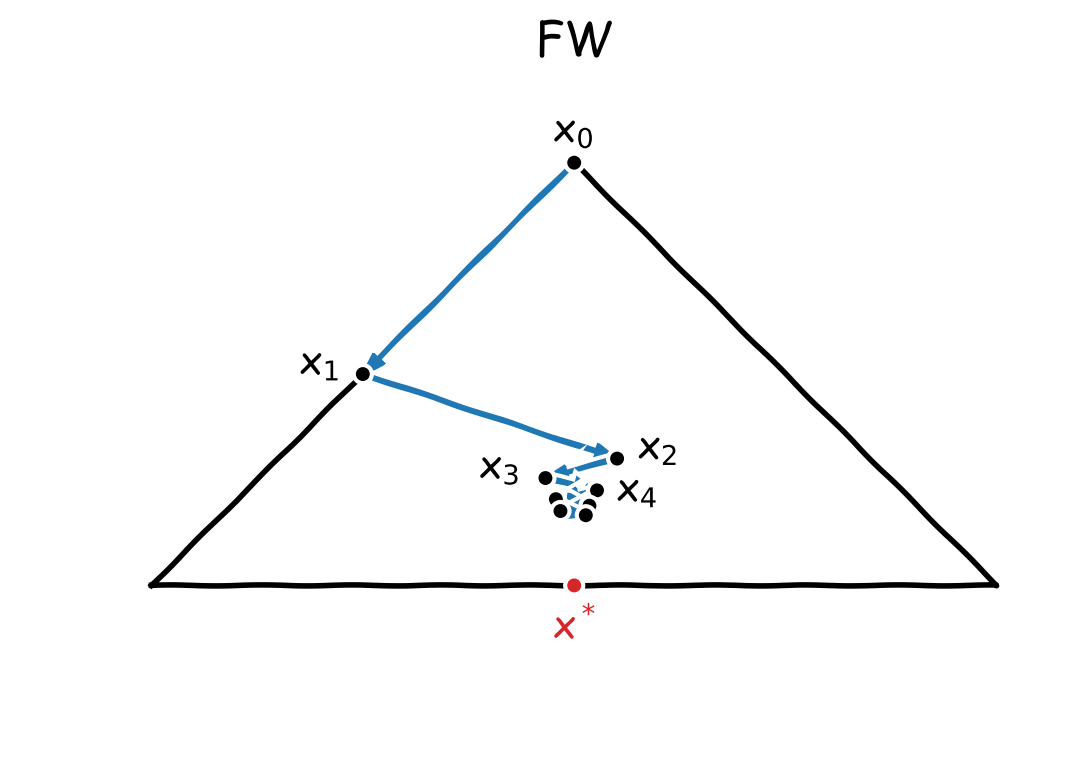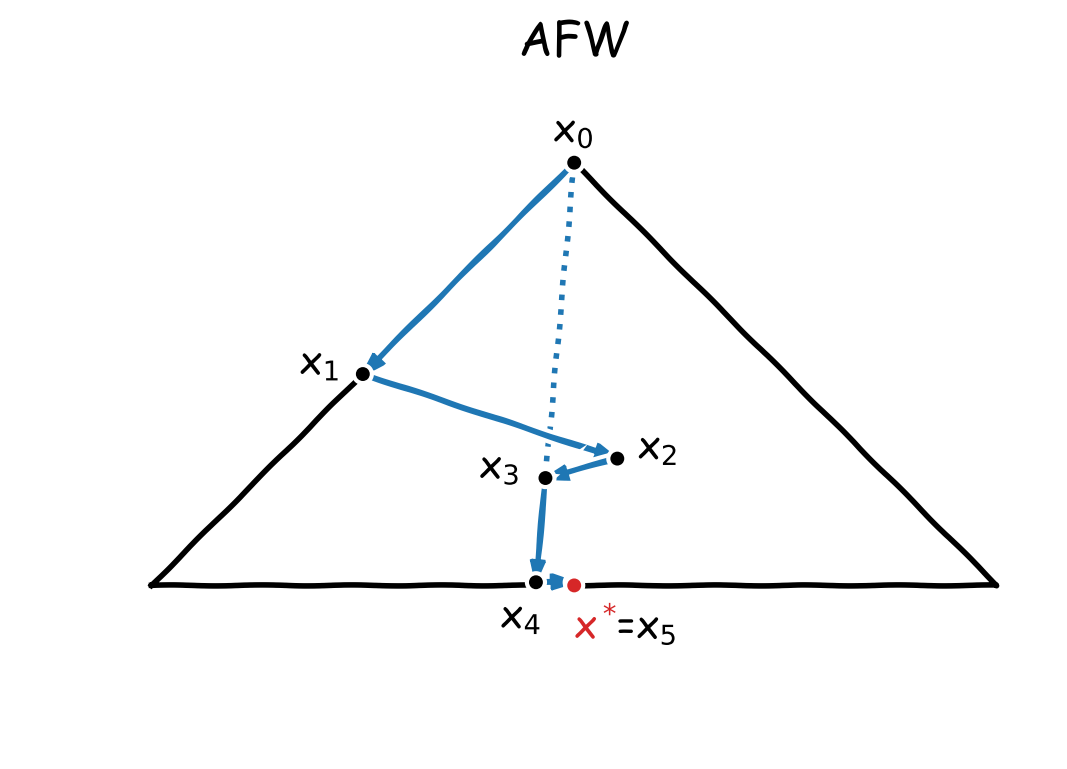Figure 1. Trajectory of the iterates of FW and AFW to minimize $f(x)=\norm{x}_2^2/2$ over the convex hull of $\setb{(-1,0),(0,1),(1,0)}$, starting from $x_0=(0,1)$. The solution is $x^\esx=(0,0)$. FW tries to reach $x^\esx$ by moving towards vertices, which is not efficient here as the directions $v_t-x_t$ become more and more orthogonal to $x^\esx-x_t$. AFW solves this issue by adding the option to move away from vertices; here, $x_4$ is obtained by moving away from $x_0$ and enables $x_5=x^\esx$.

However, the descent directions of AFW might still not be as favorable as those of gradient descent. Furthermore, in order to decide whether to move towards vertices or away from vertices and, when appropriate, to perform an away step, AFW needs to maintain the decomposition of the iterates onto $\mathcal{C}$. This can become very costly in both memory usage and computation time [GM]. Thus, we propose to directly estimate the gradient descent direction $-\nabla f(x_t)$ by sequentially picking up vertices in a matching pursuit-style [MZ]. By doing so, we can descend in directions better aligned with those of the negative gradients while still remaining projection-free.

## Boosting via gradient pursuit

At each iteration, we perform a sequence of rounds chasing the direction $-\nabla f(x_t)$. We initialize our direction estimate as $d_0\leftarrow0$. At round $k$, the residual is $r_k\leftarrow-\nabla f(x_t)-d_k$ and we aim at maximally reducing it by subtracting its maximum component among the vertex directions. We update $d_{k+1}\leftarrow d_k+\lambda_ku_k$ where $u_k\leftarrow v_k-x_t$, $v_k\leftarrow\arg\max_{v\in\mathcal{C}}\langle r_k,v\rangle$, and $\lambda_k\leftarrow\frac{\langle r_k,u_k\rangle}{\norm{u_k}^2}$: $\lambda_ku_k$ is the projection of $r_k$ onto its maximum component $u_k$. Note that this “projection” is actually closed-form and very cheap. The new residual is $r_{k+1}\leftarrow-\nabla f(x_t)-d_{k+1}=r_k-\lambda_ku_k$. We stop the procedure whenever the improvement in alignment between rounds $k$ and $k+1$ is not sufficient, i.e., whenever

\begin{align*} \frac{\langle-\nabla f(x_t),d_{k+1}\rangle}{\norm{\nabla f(x_t)}\norm{d_{k+1}}}-\frac{\langle-\nabla f(x_t),d_k\rangle}{\norm{\nabla f(x_t)}\norm{d_k}}<\delta \end{align*}

for some $\delta\in\left]0,1\right[$. In our experiments, we typically set $\delta=10^{-3}$.

We stress that $d_k$ can be well aligned with $-\nabla f(x_t)$ even when $\norm{-\nabla f(x_t)-d_k}$ is arbitrarily large: we aim at estimating the direction of $-\nabla f(x_t)$ and not the vector $-\nabla f(x_t)$ itself (which would require many more rounds). Once the procedure is completed, we use $g_t\leftarrow d_k/\sum_{\ell=0}^{k-1}\lambda_\ell$ as descent direction. This normalization ensures that the entire segment $[x_t,x_t+g_t]$ is in the feasible region $\mathcal{C}$. Hence, by updating $x_{t+1}\leftarrow x_t+\gamma_tg_t$ with a step-size $\gamma_t\in\left[0,1\right]$, we remain projection-free while descending in the direction $g_t$ better aligned with $-\nabla f(x_t)$. This is the design of our Boosted Frank-Wolfe algorithm (BoostFW). Figure 2 illustrates the procedure.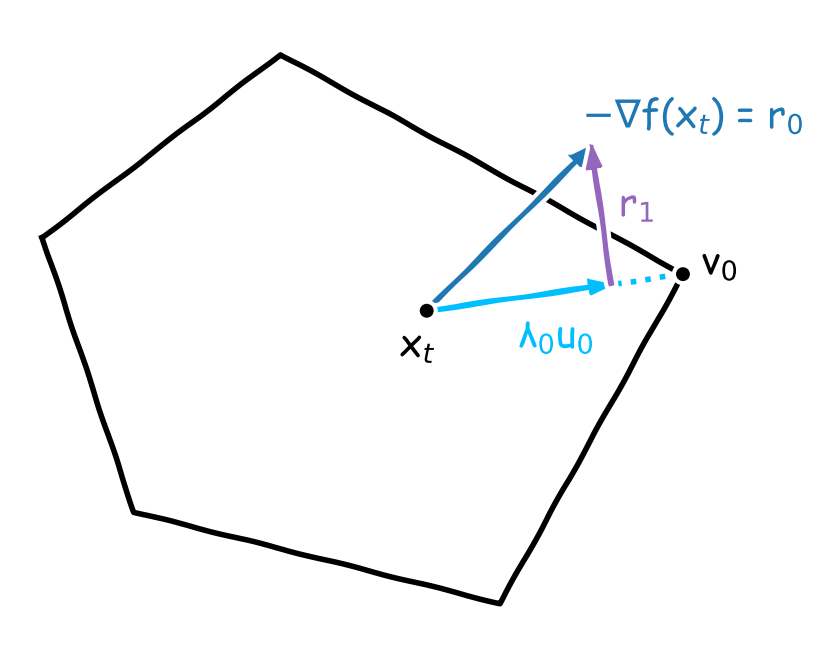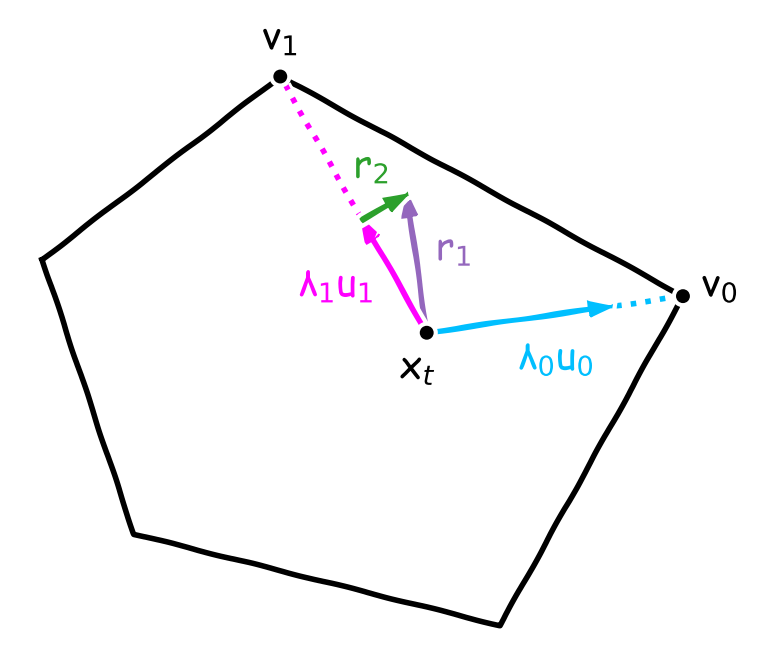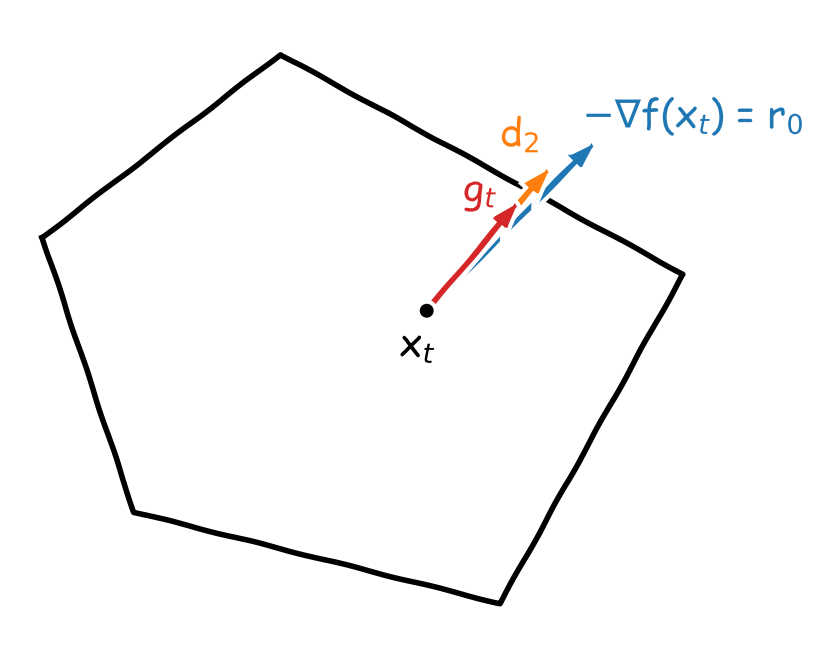Figure 2. Illustration of the gradient pursuit procedure. It builds a descent direction $g_t$ better aligned with the gradient descent direction $-\nabla f(x_t)$. We have $g_t=d_2/(\lambda_0+\lambda_1)$ where $d_2=\lambda_0u_0+\lambda_1u_1$, $u_0=v_0-x_t$, and $u_1=v_1-x_t$. Furthermore, note that $[x_t,x_t+d_2]\not\subset\mathcal{C}$ but $[x_t,x_t+g_t]\subset\mathcal{C}$. Hence, moving along the segment $[x_t,x_t+g_t]$ ensures feasibility of the new iterate $x_{t+1}$.

## Computational results

Observe that BoostFW likely performs multiple linear minimizations per iteration, while FW only performs $1$ and AFW performs $\sim2$ ($1$ for the FW vertex and $\sim1$ for the away vertex). Thus, one might wonder if the progress obtained by the gradient pursuit procedure is washed away by the higher number of linear minimizations. We conducted a series of computational experiments demonstrating that this is not the case and that the advantage is quite substantial. We compared BoostFW to AFW, DICG [GM]‚ and BCG [BPTW] on various tasks: sparse signal recovery, sparsity-constrained logistic regression, traffic assignment, collaborative filtering, and video-colocalization. Figures 3-6 show that BoostFW outperforms the other algorithms both per iteration and CPU time although it calls the linear minimization oracle more often: BoostFW makes better use of its oracle calls.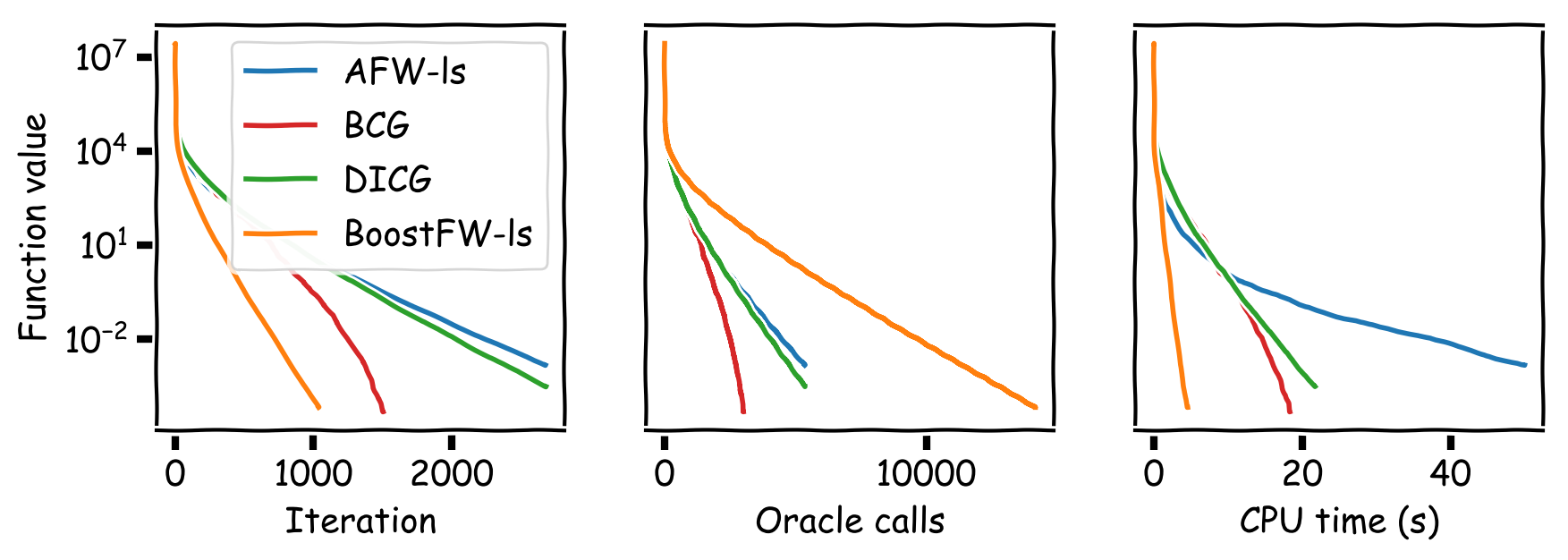Figure 3. Sparse signal recovery.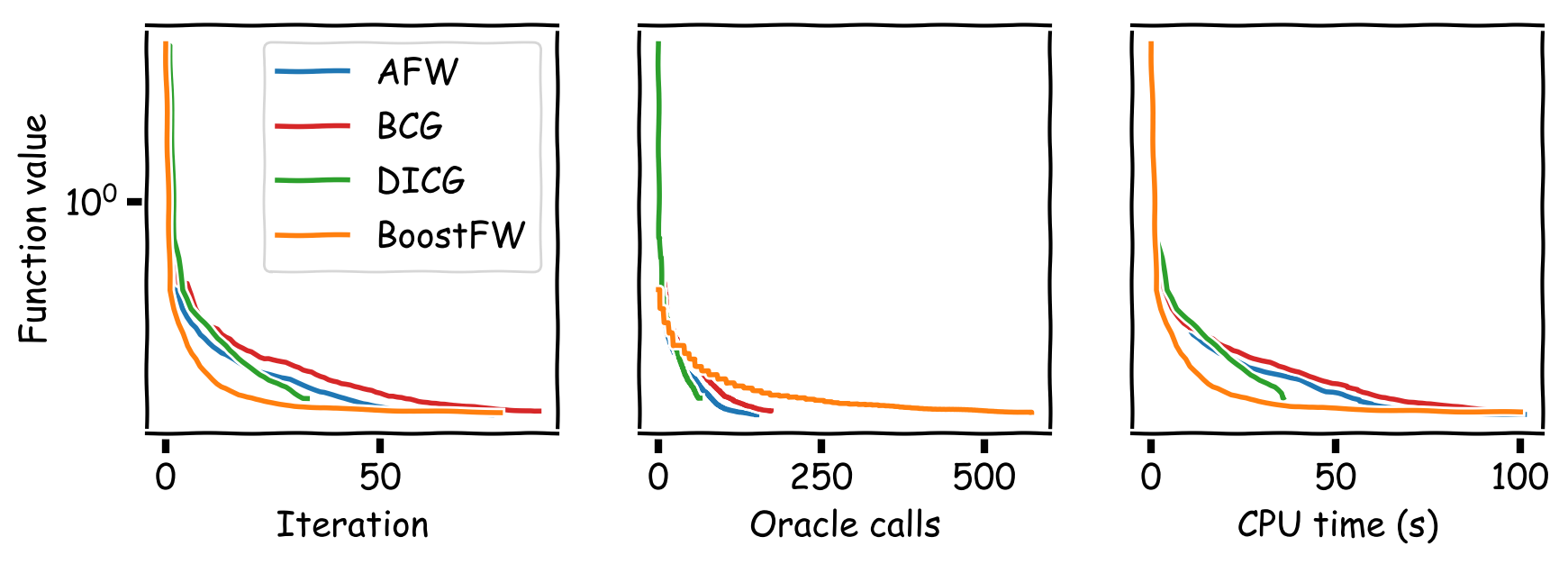Figure 4. Sparse logistic regression on the Gisette dataset.Figure 5. Traffic assignment. DICG is not applicable here.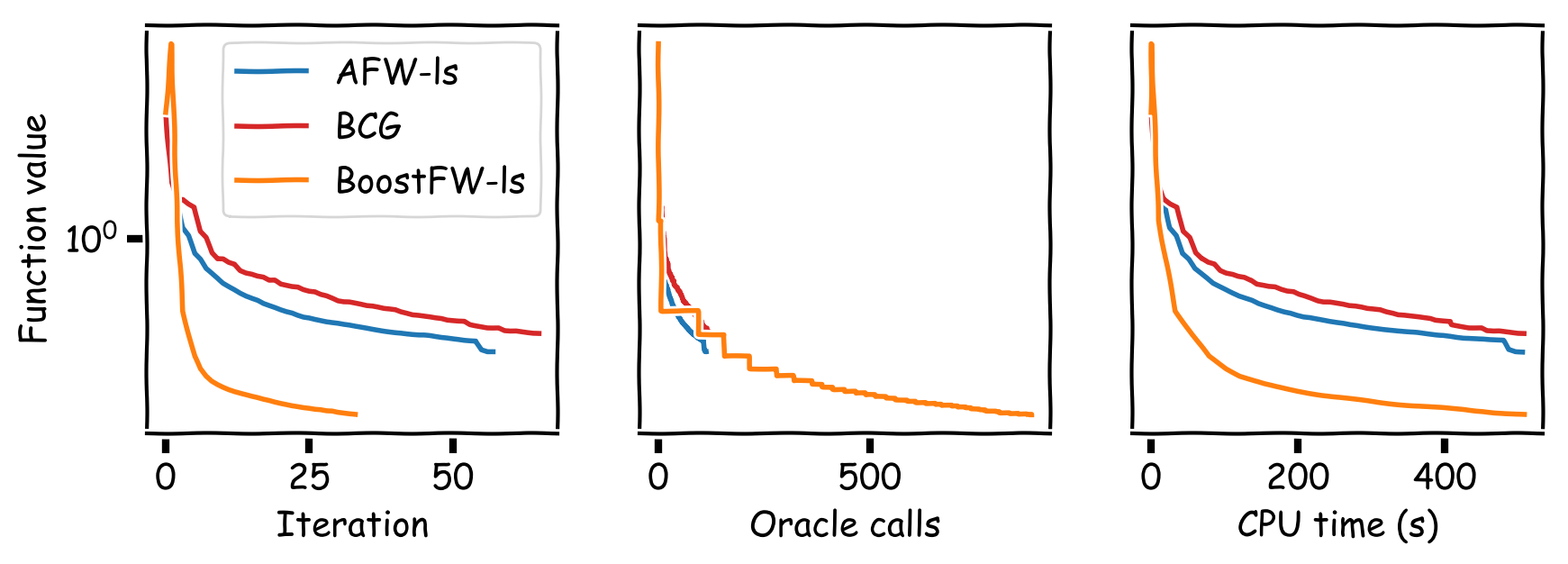Figure 6. Collaborative filtering on the MovieLens 100k dataset. DICG is not applicable here.

Lastly, we present a preliminary extension of our boosting procedure to DICG. DICG is known to perform particularly well on the video-colocalization experiment of [JTF]. The comparison is made in duality gap, in line with [GM]. Figure 7 shows promising results for BoostDICG.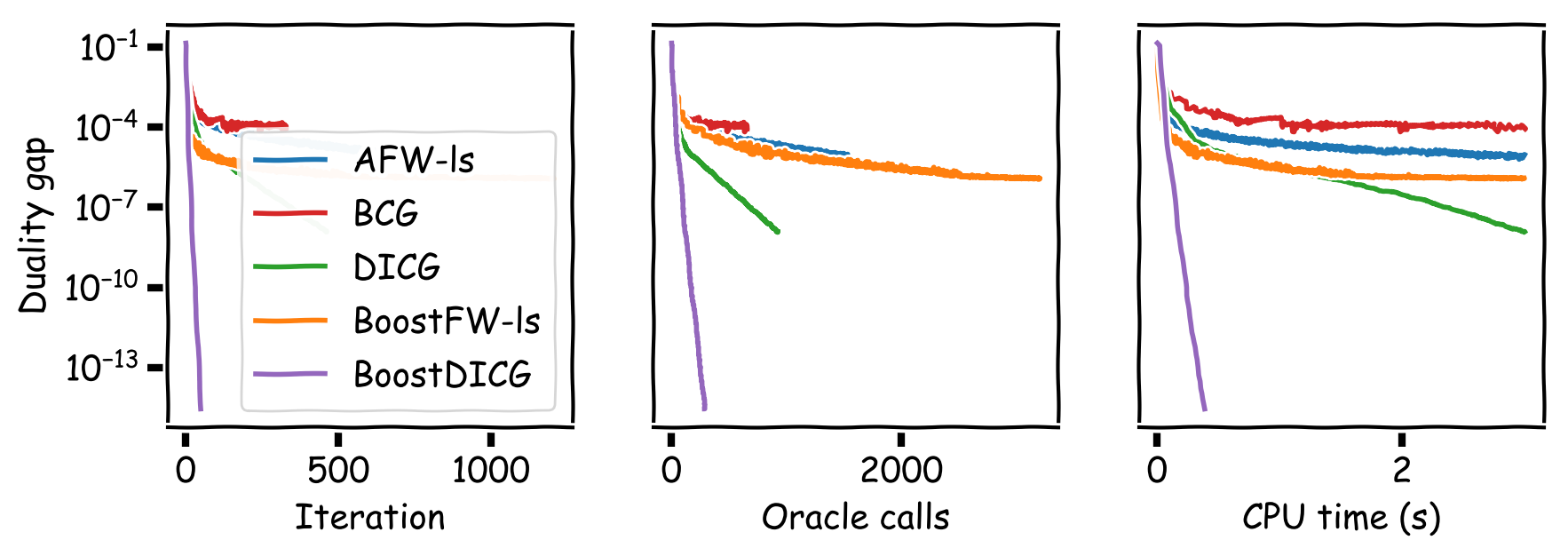Figure 7. Video-colocalization on the YouTube-Objects dataset.

[FW] Frank, M., & Wolfe, P. (1956). An algorithm for quadratic programming. Naval research logistics quarterly, 3(1‐2), 95-110. pdf

[CG] Levitin, E. S., & Polyak, B. T. (1966). Constrained minimization methods. Zhurnal Vychislitel’noi Matematiki i Matematicheskoi Fiziki, 6(5), 787-823. pdf

[W] Wolfe, P. (1970). Convergence theory in nonlinear programming. Integer and nonlinear programming, 1-36.

[LJ] Lacoste-Julien, S., & Jaggi, M. (2015). On the global linear convergence of Frank-Wolfe optimization variants. In Advances in Neural Information Processing Systems (pp. 496-504). pdf

[GM] Garber, D., & Meshi, O. (2016). Linear-memory and decomposition-invariant linearly convergent conditional gradient algorithm for structured polytopes. In Advances in Neural Information Processing Systems (pp. 1001-1009). pdf

[MZ] Mallat, S. G., & Zhang, Z. (1993). Matching pursuits with time-frequency dictionaries. IEEE Transactions on signal processing, 41(12), 3397-3415. pdf

[BPTW] Braun, G., Pokutta, S., Tu, D., & Wright, S. (2019). Blended conditional gradients: the unconditioning of conditional gradients. Proceedings of ICML. pdf

[JTF] Joulin, A., Tang, K., & Fei-Fei, L. (2014, September). Efficient image and video co-localization with frank-wolfe algorithm. In European Conference on Computer Vision (pp. 253-268). Springer, Cham. pdf